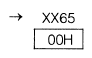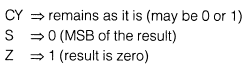Courses

# Test: Microprocessors - 2

## 20 Questions MCQ Test GATE ECE (Electronics) 2022 Mock Test Series | Test: Microprocessors - 2

Description
This mock test of Test: Microprocessors - 2 for Electronics and Communication Engineering (ECE) helps you for every Electronics and Communication Engineering (ECE) entrance exam. This contains 20 Multiple Choice Questions for Electronics and Communication Engineering (ECE) Test: Microprocessors - 2 (mcq) to study with solutions a complete question bank. The solved questions answers in this Test: Microprocessors - 2 quiz give you a good mix of easy questions and tough questions. Electronics and Communication Engineering (ECE) students definitely take this Test: Microprocessors - 2 exercise for a better result in the exam. You can find other Test: Microprocessors - 2 extra questions, long questions & short questions for Electronics and Communication Engineering (ECE) on EduRev as well by searching above.
QUESTION: 1

### If the memory chip size is 256 x 1 bits, the no. of chips required to make up 1 K (1024) bytes of memory are ____

Solution: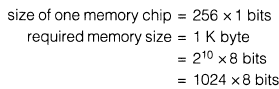Therefore required number of chips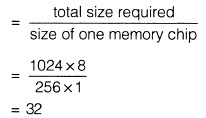QUESTION: 2

### The memory map of a 4 K(4096) byte memory chip begins at the location 2000 H. What will be the last address location on the chip.

Solution:

Address of last memory location can always be find using this formula last location’s address
= Initial address + Hexadecimal equivalent of memory size - (0001 )H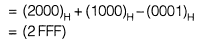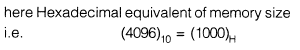QUESTION: 3

###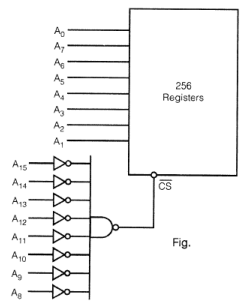Q. In the given figure address range of memory chip will be

Solution: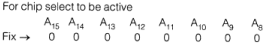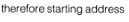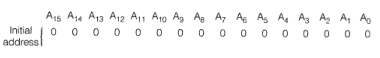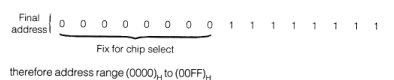QUESTION: 4Q.

If in the previous question if input to NAND gate is given directly without using NOT gate then what will be the address range.

Solution: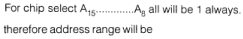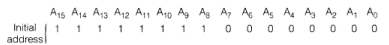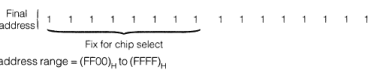QUESTION: 5

MOV B, M

the addressing mode and number of T-states required for execution of above instruction is

Solution:

This is a indirect addressing mode because the address of the data which is to be transferred to register B is given by register pair HL.
It’s execution involves 2 machine cycles opcode fetch cycle and memory read cycle and total 7-T states.

QUESTION: 6

Status of flags after execution of SUB A

Solution:

SUB A when this instruction is executed content of A is subtracted from content of A so content of accumulator after execution of this instruction is

So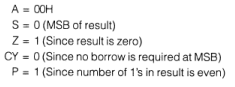QUESTION: 7

3000 : MVI D, 30H

3002 : MVI E, 01H

3004    : LDAX D

3005    : CPI 40H

3007 : HLT

When above instructions (program) is executed what will be content of A and status of flag CY and Z.

Solution: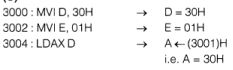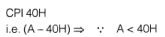therefore, CY = 1,Z = 0 and in compare instruction content of accumulator remains unchanged. Therefore content of A = (30)H.
So, correct option is (c).

QUESTION: 8

Mathematical function performed by the following instructions:

MVI A, 07H

RLC

MOV B,

A RLC

RLC

Solution: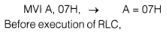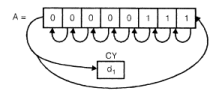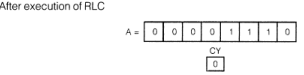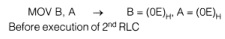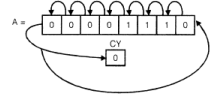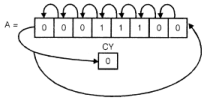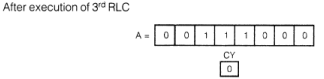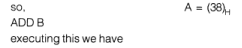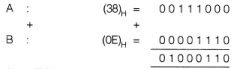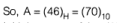Initial content of A = (07)H = (7)10. So this set of instructions multiply the content of A by 10.

QUESTION: 9

What will be content of accumulator after execution of following instructions. Also what will be sign and carry flag.

MVI A, 79H

ANI A,

FOH

RRC

RRC

RRC

RRC

Solution: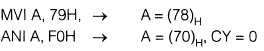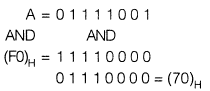Before execution first RRC,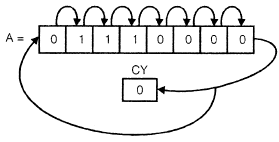QUESTION: 10

In which case RLC and RAL will give the same result

Solution:

In both cases D7 becomes carry flag, but in case of RAL carry before execution of this instruction goes to D0 while in case of RLC, D7 goes to D0. So when D7 and CY will be same then D0 in both cases will be same and result will be same.

QUESTION: 11

Specify the accumulator content and flag status (S, Z, CY) as the following instructions are executed.
XRA A

MVI B, 4AH

SUI 4FH

ANAB

HLT

Solution: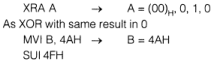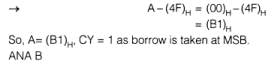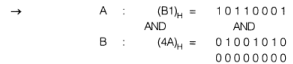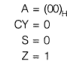QUESTION: 12

20FC : MVIB,

20H 20FE : MVI C, FFH

2100 : LDAXB

JC display

MOV A, C

OUT 01H

HLT

display : OUT 01H

Solution: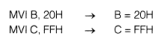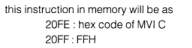Now LDXA B → load the accumulator by the content at memory location pointed by BC register pair.
i.e. loaded by content at memory location
BC = 20FFH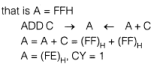Carry flag is set because carry is generated at MSB of the addition. Now conditional jump instruction JC display will be satisfied (condition is true) so control goes to display.
Therefore, output at port 01H = (FE)H.

QUESTION: 13

MVI A, 17H

LOOP:ORA A

RRC

JNC  LOOP

How many times the loop will be executed in above program,

Solution: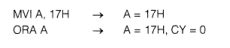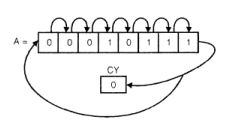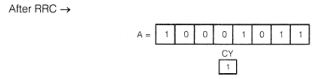the conditional jump JNC LOOP will transfer the control to LOOP if condition is true i.e. carry CY = 0. But there CY = 1 so condition is false so it comes out of loop. So loop will be executed only once.

QUESTION: 14

LXI SP, 209FH

MVI C, OOH

PUSH B P

OPPSW

RET

Q.

The function of above subroutine is

Solution: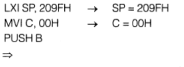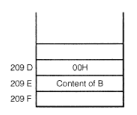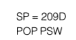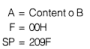So function of above subroutine is to reset all the flags.

QUESTION: 15

LXI SP, 209FH

MVI C, OOH

PUSH B P

OPPSW

RET

Q.

What will be the stack pointer after the execution of given subroutine in previous question?

Solution:

Since push instruction decreamented the stack pointer by 2. While POP operation increamented the stack pointer by 2.
Since there are one PUSH and one POP so overall effect is cancelled and content of SP remains unchanged.
SP = 209F

QUESTION: 16

Call location of TRAP the nonmaskable interrupt is

Solution:

For finding call location we treat it as RST 4.5.
So, call location = Hexadecimal equivalent of 8 x4.5
= (36)10 = (0024)H

QUESTION: 17

Given:
MVI A, OEH

SIM

In above subroutine which one of the following interrupt is enabled?

Solution: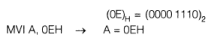SIM instruction interprets the accumulator content as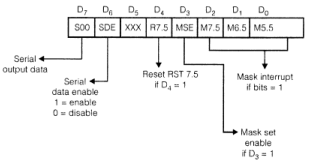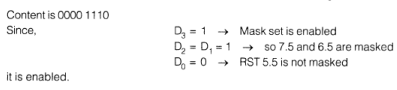QUESTION: 18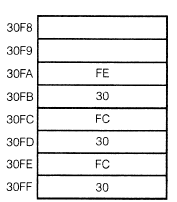Initially the memory is given as above.

LX I SP, 30FAH

POP D

LHLD 30FCH

SPHL

LDAXD

MOVC, A

INXD

LDAXD

MOV B, A

HLT

After the execution of following program which of the following is true.

Solution: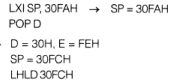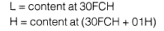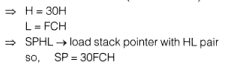LDAX D → load accumualtor indirect by the content at memory location pointed by DE pair.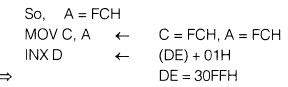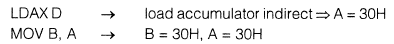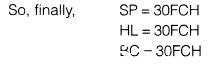QUESTION: 19

LX I B,2384H
LOOP:    DCX B    Clock frequency
MOV A, C               is 2 MHz.
ORAB

JNZ LOOP

The delay provided by above set of instructions, neglecting delay due to LXI instruction is    _____________msec

Solution:

The loop includes four instructions DCX, MOV, ORA, and JNZ takes 24 clocks (6 + 4 + 4 + 10) for execution. The loop is repeated 2384H times. Which is converted to decimal as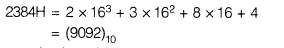it will be executed 2383H times completely and 1 time partially as condition becomes false f = 2 MHz.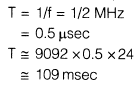QUESTION: 20

Identify the contents of the memory location XX65H and the status of the flags S, Z, CY when the instruction INR M is executed.

LX I H,XX65H

MVI M, FFH

INR M

HLT

Solution:

LXI H, XX65H → HL = XX65H MVI M, FFH
Memory location pointed by HL pair will be loaded by the immediate data that is data at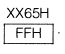INR M    data at memory location pointed by HL pair is increamented by 1 so it becomes